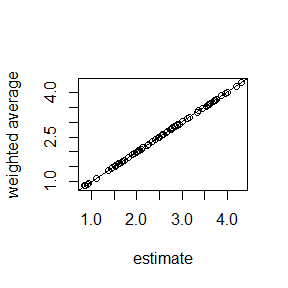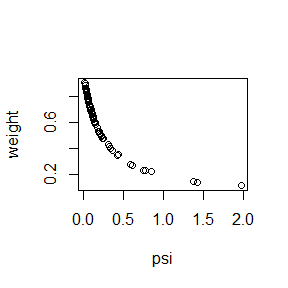Basic area-level model

The basic area-level model (Fay and Herriot 1979; Rao and Molina 2015) is given by $y_i | \theta_i \stackrel{\mathrm{ind}}{\sim} {\cal N} (\theta_i, \psi_i) \,, \\ \theta_i = \beta' x_i + v_i \,,$ where $$i$$ runs from 1 to $$m$$, the number of areas, $$\beta$$ is a vector of regression coefficients for given covariates $$x_i$$, and $$v_i \stackrel{\mathrm{ind}}{\sim} {\cal N} (0, \sigma_v^2)$$ are independent random area effects. For each area an observation $$y_i$$ is available with given variance $$\psi_i$$.

First we generate some data according to this model:

m <- 75L  # number of areas
df <- data.frame(
area=1:m,      # area indicator
x=runif(m)     # covariate
)
v <- rnorm(m, sd=0.5)    # true area effects
theta <- 1 + 3*df$x + v # quantity of interest psi <- runif(m, 0.5, 2) / sample(1:25, m, replace=TRUE) # given variances df$y <- rnorm(m, theta, sqrt(psi))

A sampler function for a model with a regression component and a random intercept is created by

library(mcmcsae)
model <- y ~ reg(~ 1 + x, name="beta") + gen(factor = ~iid(area), name="v")
sampler <- create_sampler(model, sigma.fixed=TRUE, Q0=1/psi, linpred="fitted", data=df)

The meaning of the arguments used here is as follows:

• the first argument is a formula specifying the response variable and the linear predictor part of the model
• sigma.fixed=TRUE signifies that the observation level variance parameter is fixed at 1. In this case it means that the variances are known and given by psi.
• the function expects precisions rather than variances, and with Q0=1/psi the precisions are set to the vector 1/psi.
• linpred="fitted" indicates that we wish to obtain samples from the posterior distribution for the vector $$\theta$$ of small area means.
• data is the data.frame in which variables used in the model specification are looked up.

An MCMC simulation using this sampler function is then carried out as follows:

sim <- MCMCsim(sampler, store.all=TRUE, verbose=FALSE)

A summary of the results is obtained by

(summ <- summary(sim))
## llh_ :
##     Mean       SD  t-value     MCSE    q0.05     q0.5    q0.95    n_eff    R_hat
##  -33.118    5.961   -5.556    0.144  -43.526  -32.845  -23.603 1709.801    1.001
##
## linpred_ :
##    Mean    SD t-value    MCSE q0.05 q0.5 q0.95 n_eff R_hat
## 1  1.91 0.419    4.56 0.00874  1.21 1.91  2.58  2297 1.001
## 2  2.65 0.230   11.56 0.00434  2.28 2.65  3.04  2804 1.000
## 3  2.91 0.271   10.75 0.00507  2.46 2.91  3.34  2845 1.000
## 4  3.46 0.440    7.87 0.00937  2.72 3.48  4.17  2208 1.000
## 5  1.62 0.196    8.25 0.00369  1.29 1.62  1.93  2810 1.001
## 6  4.00 0.183   21.89 0.00341  3.70 4.00  4.30  2866 1.000
## 7  2.75 0.442    6.22 0.00871  2.02 2.75  3.45  2573 1.002
## 8  2.68 0.171   15.68 0.00312  2.41 2.68  2.97  3000 0.999
## 9  1.92 0.220    8.71 0.00408  1.56 1.92  2.28  2910 0.999
## 10 2.68 0.402    6.68 0.00797  2.01 2.68  3.34  2538 1.000
## ... 65 elements suppressed ...
##
## beta :
##             Mean    SD t-value    MCSE q0.05 q0.5 q0.95 n_eff R_hat
## (Intercept) 1.10 0.150    7.38 0.00837 0.861 1.11  1.34   320     1
## x           2.86 0.255   11.20 0.01308 2.444 2.85  3.28   381     1
##
## v_sigma :
##     Mean       SD  t-value     MCSE    q0.05     q0.5    q0.95    n_eff    R_hat
## 4.68e-01 6.41e-02 7.30e+00 1.84e-03 3.71e-01 4.63e-01 5.78e-01 1.21e+03 1.00e+00
##
## v :
##       Mean    SD t-value    MCSE   q0.05    q0.5  q0.95 n_eff R_hat
## 1  -0.1702 0.414  -0.411 0.00815 -0.8729 -0.1717  0.491  2585     1
## 2   0.0745 0.235   0.316 0.00501 -0.3132  0.0723  0.467  2207     1
## 3  -0.2206 0.274  -0.806 0.00570 -0.6671 -0.2189  0.223  2304     1
## 4  -0.4236 0.425  -0.997 0.00805 -1.1586 -0.4032  0.236  2783     1
## 5   0.3549 0.224   1.585 0.00778 -0.0186  0.3488  0.722   828     1
## 6   0.0547 0.222   0.246 0.00753 -0.3083  0.0571  0.419   872     1
## 7   0.0684 0.438   0.156 0.00860 -0.6437  0.0753  0.776  2590     1
## 8   0.1355 0.184   0.738 0.00471 -0.1582  0.1335  0.447  1520     1
## 9  -0.5569 0.225  -2.479 0.00466 -0.9301 -0.5554 -0.192  2328     1
## 10  0.2493 0.400   0.623 0.00782 -0.4079  0.2492  0.913  2622     1
## ... 65 elements suppressed ...

In this example we can compare the model parameter estimates to the ‘true’ parameter values that have been used to generate the data. In the next plots we compare the estimated and ‘true’ random effects, as well as the model estimates and ‘true’ estimands. In the latter plot, the original ‘direct’ estimates are added as red triangles.

plot(v, summ$v[, "Mean"], xlab="true v", ylab="posterior mean"); abline(0, 1) plot(theta, summ$linpred_[, "Mean"], xlab="true theta", ylab="estimated"); abline(0, 1)
points(theta, df$y, col=2, pch=2)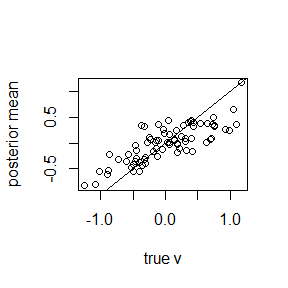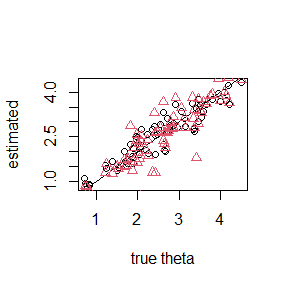We can compute model selection measures DIC and WAIC by compute_DIC(sim) ## DIC p_DIC ## 112.86192 46.62546 compute_WAIC(sim, show.progress=FALSE) ## WAIC1 p_WAIC1 WAIC2 p_WAIC2 ## 87.84794 21.61157 108.87962 32.12741 Posterior means of residuals can be extracted from the simulation output using method residuals. Here is a plot of (posterior means of) residuals against covariate $$x$$: plot(df$x, residuals(sim, mean.only=TRUE), xlab="x", ylab="residual"); abline(h=0)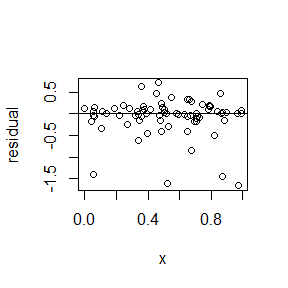A linear predictor in a linear model can be expressed as a weighted sum of the response variable. If we set compute.weights=TRUE then such weights are computed for all linear predictors specified in argument linpred. In this case it means that a set of weights is computed for each area.

sampler <- create_sampler(model, sigma.fixed=TRUE, Q0=1/psi,
linpred="fitted", data=df, compute.weights=TRUE)
sim <- MCMCsim(sampler, store.all=TRUE, verbose=FALSE)

Now the weights method returns a matrix of weights, in this case a 75 $$\times$$ 75 matrix $$w_{ij}$$ holding the weight of direct estimate $$i$$ in linear predictor $$j$$. To verify that the weights applied to the direct estimates yield the model-based estimates we plot them against each other. Also shown is a plot of the weight of the direct estimate for each area in the predictor for that same area, against the variance of the direct estimate.

plot(summ$linpred_[, "Mean"], crossprod(weights(sim), df$y),
xlab="estimate", ylab="weighted average")
abline(0, 1)
plot(psi, diag(weights(sim)), ylab="weight")